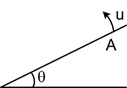A body is projected from point A on an inclined plane having inclination θ with horizontal with velocity u in a direction perpendicular to the inclined plane as shown in figure. Then range of the projectile on inclined plane is

# A body is projected from point A on an inclined plane having inclination θ with horizontal with velocity u in a direction perpendicular to the inclined plane as shown in figure. Then range of the projectile on inclined plane is1. A

$\frac{2{\mathrm{u}}^{2}}{\mathrm{g}}\mathrm{tan\theta }.\mathrm{sec\theta }$

2. B

$\frac{{\mathrm{u}}^{2}}{\mathrm{g}}\mathrm{sin}2\mathrm{\theta }.\mathrm{cos\theta }$

3. C

$\frac{2{\mathrm{u}}^{2}}{\mathrm{g}}\mathrm{sec\theta }$

4. D

$\frac{{\mathrm{u}}^{2}}{\mathrm{g}}\mathrm{sin\theta }$

Fill Out the Form for Expert Academic Guidance!l

+91

Live ClassesBooksTest SeriesSelf Learning

Verify OTP Code (required)

### Solution:

Considering X axis along inclined plane and Y axis perpendicular to inclined plane.

$\begin{array}{l}{\mathrm{T}}_{\mathrm{i}}=\mathrm{Time}\text{\hspace{0.17em}}\mathrm{of}\text{\hspace{0.17em}}\mathrm{flight}\text{\hspace{0.17em}}\mathrm{on}\text{\hspace{0.17em}}\mathrm{inclined}\text{\hspace{0.17em}\hspace{0.17em}}\mathrm{plane}\\ =\frac{2\mathrm{u}}{\mathrm{gcos\theta }}\\ \therefore \text{\hspace{0.17em}}{\mathrm{R}}_{\mathrm{i}}=\frac{1}{2}\left(\mathrm{gsin\theta }\right){{\mathrm{T}}_{\mathrm{i}}}^{2}=\frac{1}{2}\left(\mathrm{gsin\theta }\right)\left(\frac{4{\mathrm{u}}^{2}}{{\mathrm{g}}^{2}{\mathrm{cos}}^{2}\mathrm{\theta }}\right)\\ {\mathrm{R}}_{\mathrm{i}}=\frac{2{\mathrm{u}}^{2}}{\mathrm{g}}\mathrm{tan\theta }.\mathrm{sec\theta }\end{array}$+91

Live ClassesBooksTest SeriesSelf Learning

Verify OTP Code (required)